# Your Lopsided Model is Out to Get You

For classification problems I argue one of the biggest steps you can take to improve the quality and utility of your models is to prefer models that return scores or return probabilities instead of classification rules. Doing this also opens a second large opportunity for improvement: working with your domain experts to find new variables to lower the variance in prediction on your weakest outcome category.

In this note we will show the problems of unbalanced or lopsided classification models, and suggest how to use this variance criticism as a tool for improvement.

## Introduction

I believe that many experienced data scientists have run into the following problem. You build a scoring model or probability scoring model and pick an “obviously best example” to show that the score predicts outcome. The model then kicks you in the teeth, and shows the outcome that is not supposed to happen. For example: you build a credit model to predict if a transaction is safe, you take the example with highest safety score, and this example turns out to be fraud.

I used to think this was just an example of the so-called “law of small numbers”: small samples have good chances of showing weird behavior. However, I now feel there are some common modeling flaws that actually can make this sort of malfunction very likely.

### Set Up

I am going to show the following.

Unless one controls for prediction variance conditioned on outcome, one is quite likely to see model predictions that are wrong on one side of a model scoring range.

For example, a seemingly good model where high scores predict good transactions may assign highest scores to some fraudulent transactions. We can call this “the model going insane at one of the ends.”

This is an understood issue related to the un-desirable presence of non-constant variance of the prediction when conditioned on the ground-truth outcome. Roughly we can think of this as heteroscedasticity, but in a signal detection context. This issue is a reason why equal variance is often assumed (or insisted on) in ROC (Receiver Operating Characteristic plot) frameworks (reference: James P. Egan, Signal Detection Theory and ROC Analysis, Academic Press, 1975).

### Our Example

Let’s make this more specific through a concrete example.

We are building a model to sort possible fraudulent transactions from good ones. We are in the nice situation where our model score is a normally distributed number in what is called “link space” (the logit of a probability, or the logarithm of an odds ratio). High scores are indicative of non-fraudulent transactions and low scores are indicative of fraudulent transactions. And we are in a nice case where fraudulent transactions are rare.

Let’s use `R` to set up a synthetic example with these properties.

``````# attach our packages
library(ggplot2)
library(WVPlots)
library(rquery)``````
``````# specify parameters
fraud_prevalence <- 0.01
data_size = 10000
mean_good <- 0
sd_good <- 1
mean_fraud <- -1.5
sd_fraud <- 2``````
``````# generate example data
set.seed(2020)

examples <- rbind(
data.frame(  # fraud examples have one normal distribution
goodness_score = rnorm(
n = round(fraud_prevalence*data_size),
mean = mean_fraud,
sd = sd_fraud),
ground_truth = 'fraud',
stringsAsFactors = FALSE),
data.frame(  # non-fraud examples have another normal distribution
goodness_score = rnorm(
n = round((1-fraud_prevalence)*data_size),
mean = mean_good,
sd = sd_good),
ground_truth = 'good',
stringsAsFactors = FALSE)
)``````

Let’s confirm the mean score for good examples is higher than the mean-score for fraudulent examples.

``````examples %.>%
aggregate(goodness_score ~ ground_truth, data = ., FUN = mean) %.>%
rename_columns(., 'mean_goodness_score' := 'goodness_score') %.>%
knitr::kable(.)``````
ground_truth mean_goodness_score
fraud -1.2822164
good -0.0113797

The above might be all one checks for in a linear discriminant framework. Obviously, we should look at a lot more.

And now let’s exhibit the problem: the item with highest goodness score is an example of fraud!

``````examples %.>%
.[.\$goodness_score >= max(.\$goodness_score), ] %.>%
knitr::kable(.)``````
goodness_score ground_truth
64 4.903264 fraud

This is very embarrassing. The example with the highest “good” score is fraud. And “fraud” is the rare class.

``````table(ground_truth = examples\$ground_truth) %.>%
knitr::kable(.)``````
ground_truth Freq
fraud 100
good 9900

How did it even sneak in? Well that is what fraud does.It is good that we spot-checked this. Hopefully we checked this before the model went into production, or before our boss performed such a spot-check.

### Diagnosis

Let’s look at the data. First we plot the distribution of model scores conditioned on outcome.

``````ggplot(
data = examples,
mapping = aes(x = goodness_score, fill = ground_truth)) +
geom_histogram(bins = 50) +
facet_wrap(~ ground_truth, ncol = 1, scales = 'free_y') +
scale_fill_brewer(palette = "Dark2") +
ggtitle("Distribution of model score conditioned on ground truth") ``````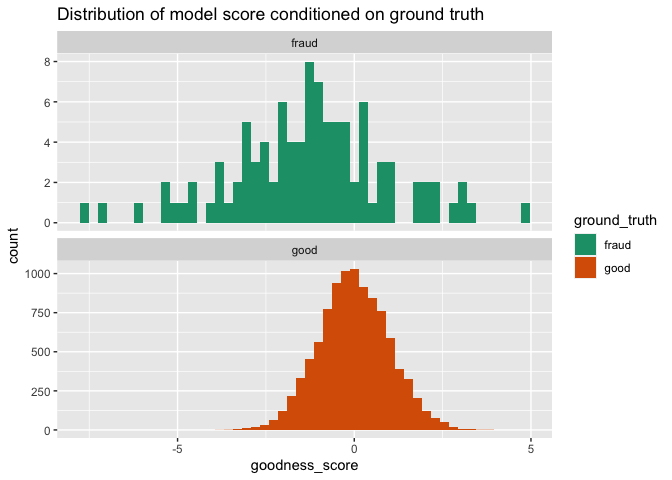p>We now see the source of the problem. The fraudulent transactions do have a lower mean, but they also a higher standard deviation (or variance).

``````examples %.>%
aggregate(goodness_score ~ ground_truth, data = ., FUN = sd) %.>%
rename_columns(., 'sd_goodness_score' := 'goodness_score') %.>%
knitr::kable(.)``````
ground_truth sd_goodness_score
fraud 2.2385836
good 0.9955967

Obviously emprical standard deviations will never exactly match, but these are pretty far off.

We will show that this means the fraudulent transactions dominate both the lowest and highest regions of model score! This isn’t just a small-data problem. This (simulated) classifier has a flaw that few data scientists still check for: the model prediction has different variances for different values of the ground truth. This is a model pathology that we should look for and try and fix.

#### More diagnostics

At this point it should be easy to argue that visualizing your results is very important. Having some canned or ready-to-go diagnostic plots makes this much easier, and makes it more likely one will take the time to look. Let’s try a few such plots from the WVPlots package.

On a double density plot the mis-matched variances are much easier to see.

``````DoubleDensityPlot(
examples,
xvar = 'goodness_score',
truthVar = 'ground_truth',
truth_target = 'good',
title = "Density of model predictions conditioned on ground truth")``````On a traditional ROC plot the flaw is the non-convex kink where the model dives under the `y=x` line.

``````ROCPlot(
examples,
xvar = 'goodness_score',
truthVar = 'ground_truth',
truthTarget = 'good',
title = "ROC plot")``````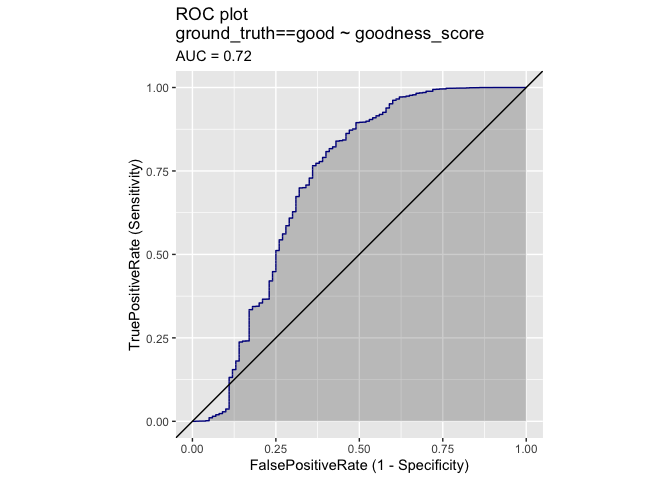Modern data scientists know to look for dips below the `y=x` line. Looking for non-convexities (or “improper ROC plots”) is a now under-taught precaution. In signal detection parlance the model is considered inadmissible or inoperable in regions where the actual or empirical ROC curve is below its convex hull (reference: James P. Egan, Signal Detection Theory and ROC Analysis, Academic Press, 1975).

``````ROCPlot(
examples,
xvar = 'goodness_score',
truthVar = 'ground_truth',
truthTarget = 'good',
title = "ROC plot with convex hull")``````In this case we see the model is not to be trusted in the region of `1-Specificity` less than 0.3 or of `Sensitivity` below `0.7`.

## The Cause

Now that we see the problem I want to show that this isn’t a small-data chance-driven fluke. This is an issue arising from the mis-matched standard deviations (or variances) of the predictions when conditioned on ground truth.

To get away from discrete empirical data, let’s look at the ideal densities that generated our empirical data.

``````# points to plot density at
goodness_score <- seq(-5, 5, by = 0.01)``````
``````# generate the theoretical densities
d_at_variance <- rbind(
data.frame(
goodness_score = goodness_score,
log_density = dnorm(
goodness_score,
mean = mean_good,
sd = sd_good,
log = TRUE),
distribution = 'dist_good',
stringsAsFactors = FALSE
),
data.frame(
goodness_score = goodness_score,
log_density = dnorm(
goodness_score,
mean = mean_fraud,
sd = sd_fraud,
log = TRUE),
distribution = 'dist_fraud',
stringsAsFactors = FALSE
))
d_at_variance\$density <- exp(d_at_variance\$log_density)``````
``````# plot the densities
ggplot(
data = d_at_variance,
mapping = aes(
x = goodness_score,
y = density,
color = distribution)) +
geom_line() +
scale_color_brewer(palette = "Dark2") +
ggtitle("Two densities with different variances")``````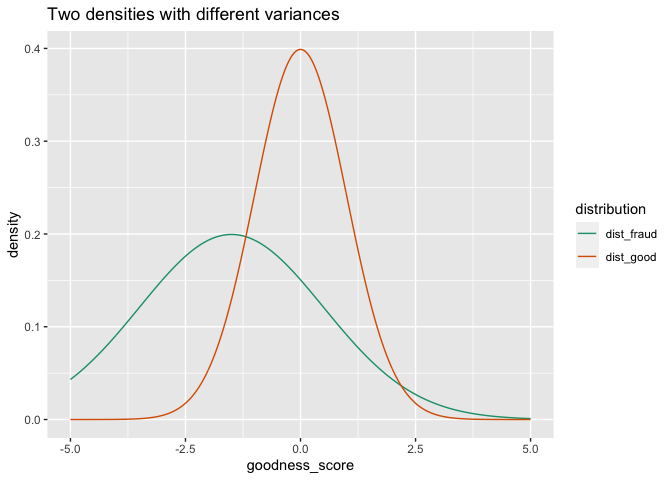Notice the densities show the same flaw we saw in the empirical simulated data: the fraud cases dominate the high-scoring region of the density plot. Visually this is seen as the `dist_fraud` density is above the `dist_good` density on both sides of the graph. Or: the density plots cross twice. This is due to the larger variance seen in the predictions conditioned on the ground truth having a value of “fraud.”

Where these crossings happen in practice depends on the prevalence, which is not shown in these graphs, but the crossing happen for all proposed prevalences.

The crossings are even clearer if we plot log-densities, which for normal distributions are just downward opening parabola.

``````ggplot(
data = d_at_variance,
mapping = aes(
x = goodness_score,
y = log_density,
color = distribution)) +
geom_line() +
scale_color_brewer(palette = "Dark2") +
ggtitle("Two log-densities with different variances")``````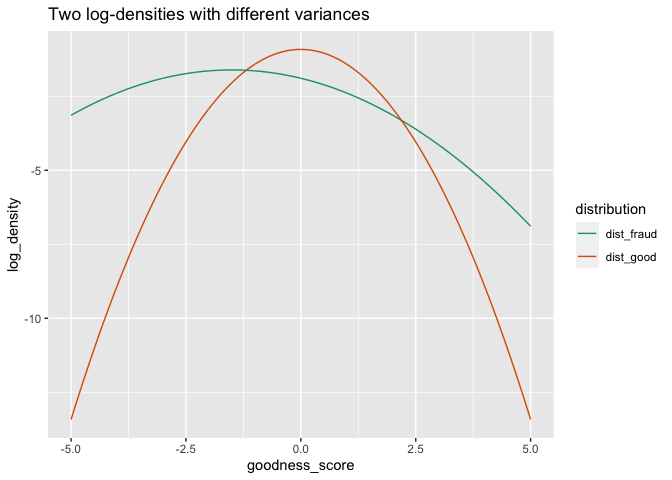And this is the problem.Two parabolas arising from normals with different variances cross each other twice, not once. That means one of the parabolas (the “good” density) is only highest for a finite interval of model scores, and the other (the “fraud” density) is highest on both ends of the plot.

This means this model score is not a monotone predictor of the ground truth, which is something we implicitly assume when we convert such a model score into a decision rule by comparing a decision threshold.

As we have said, this pathology remains present even if we re-scale the densities to reflect prevalence.

### The Failing Intuition

The intuition that is failing us is: we think of model scores as having the same variance for each ground-truth class. But in practice we rarely check for this, and very rarely teach how to establish this. However in signal detection theory, the equal variance model was a central assumption to be enfornced and probably checked (reference: James P. Egan, Signal Detection Theory and ROC Analysis, Academic Press, 1975).

When variances/standard-deviations match then we get a score that induces proper intervals. One ground truth class dominates on one side of a prediction score threshold, and the other ground truth class dominates on the other side of the same prediction score threshold. The plots in such a situation look like the following.

``````# generate simulated density where both conditional
# distributions hare the same variance or standard deviation
d_same <- rbind(
data.frame(
goodness_score = goodness_score,
log_density = dnorm(
goodness_score,
mean = mean_good,
sd = sd_good,
log = TRUE),
distribution = 'dist_good',
stringsAsFactors = FALSE
),
data.frame(
goodness_score = goodness_score,
log_density = dnorm(
goodness_score,
mean = mean_fraud,
sd = sd_good,
log = TRUE),
distribution = 'dist_fraud',
stringsAsFactors = FALSE
))
d_same\$density <- exp(d_same\$log_density)``````
``````# plot the idea case
ggplot(
data = d_same,
mapping = aes(
x = goodness_score,
y = density,
color = distribution)) +
geom_line() +
scale_color_brewer(palette = "Dark2") +
ggtitle("Two densities with same variance")``````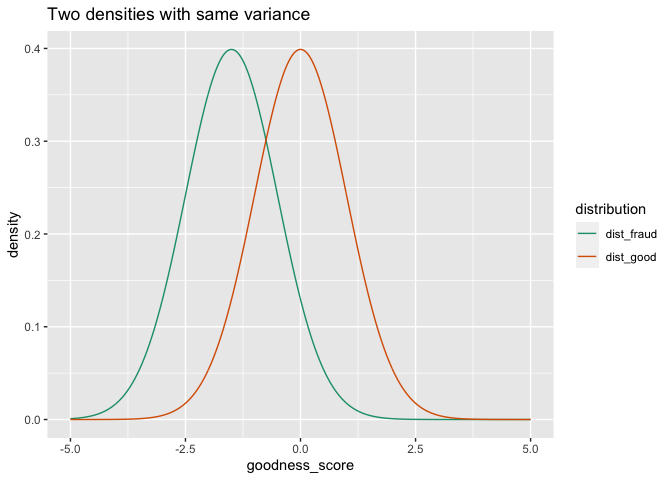``````ggplot(
data = d_same,
mapping = aes(
x = goodness_score,
y = log_density,
color = distribution)) +
geom_line() +
scale_color_brewer(palette = "Dark2") +
ggtitle("Two log-densities with same variance")``````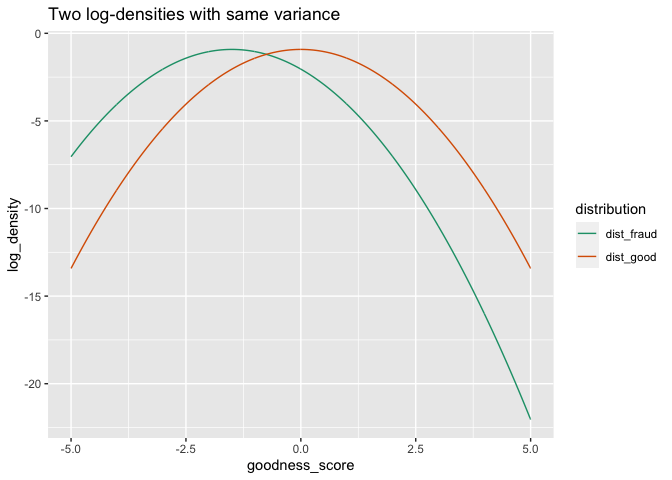Parabolas with the same curvature are either disjoint, coincident, or cross each other exactly once. There are no reversals in trend.

### Why Do Things Seem to Work?

If mismatching variances are so bad, why does modeling routinely work well?One reason is: we tend to pick models by optimization. If we are using an optimization objective function such as deviance or cross-entropy then these out of order predictions are the ones most strongly penalized against. For deviance / cross-entropy, being wrong on extreme scores costs much more than being wrong on intermediate scores. So deviance-based procedures likely balance the variances as one of their consequences. Other objective functions may not have similar effects.

So the inspection of conditional variances may be more important when using objectives other than deviance or cross-entropy.

## The Intermediate Conclusion

Models getting the highest or lowest scoring examples systematically wrong is not always due to chance. In fact this flaw can be forced when we have the pathology that our model’s scores have difference standard-deviations or variances when conditioned on ground truth. Not checking these conditional standard deviations is a bad, but likely a common practice.

## The Fix

To mitigate the above pathology we can add the following procedure to our modeling practice:

Introduce more diverse features relevant to the ground truth class with the larger variance.

The idea is: for a large class of models (including Naive Bayes, logistic regression, and possibly tree-based ensemble methods such as gradient boosting and random forest) we can think of the prediction score as hopefully being a sum of small somewhat independent contributions from variables or from conjunctions/interactions of variables. If such holds, then we expect adding more diverse variables relevant to a ground truth class should help decrease the variance in model predictions seen for that class.

Or in less technical, and more domain friendly terms.

The prediction density with the larger variance is the one your model understands the least. Use that criticism to drive improvements.

We suggest: try and bolster the model’s understanding by introducing more diverse variables related to the large variance class.

In our example fraud had higher variance, we can take this as evidence that we have more useful variables describing the behavior of non-fraudulent transactions, and fewer describing froud. Our quickest avenue for model improvement would be to sample some examples of fraud and look for common features to add to our model.

## The Actual Conclusion

The double density plot contains a lot of diagnostic information. For example: seeing different standard deviations or variances is a warning of one type of a common model pathology that can cause an undesirable reversal of model signal. Eliminating this issue by introducing more diverse variables relevant to the high-variance class can be a constructive path to a fundamentally better model. The plots are giving hints as to what sore of variables one should wish for, or what to ask domain expert partners to look into.### jmount

Data Scientist and trainer at Win Vector LLC. One of the authors of Practical Data Science with R.